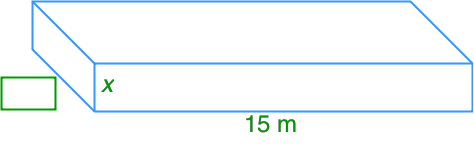# Dividing polynomials by monomials

### Dividing polynomials by monomials

This section will teach us how to divide a polynomial (more than one term) by a monomial (one term only). We will use a model to help us on the division. We will then try to solve the questions without using the model. At the end, we will look at some of the related word problems.

#### Lessons

• Introduction
a)
How to divide polynomials by monomials?

• 1.
Divide by using a model.
a)
$\frac{{6{x^2} - 24x}}{{3x}}$

b)
$\frac{{ - 5{x^2} - 7x}}{{ - x}}$

• 2.
Divide.
a)
$\frac{{4{x^2} + 12xy}}{{2x}}$

b)
$\frac{{3.5{x^2} + 2.1x}}{{7x}}$

c)
$\frac{{ - {x^2} - 1.8xy}}{{6x}}$

d)
$\frac{{ - 18{x^2} - 9x + 0.3}}{{0.3}}$

• 3.
The volume of the diagram below is $45{x^2} + 3x$.a)
Write the polynomial expression for the width of the diagram.

b)
If x = 3 m, calculate the width and the volume.